upm  1.7.1 Sensor/Actuator repository for libmraa (v2.0.0)
MPL3115A2 Class Reference

API for the MPL3115A2 Atmospheric Pressure Sensor. More...

## Detailed Description

Freescale Semiconductor* MPL3115A2 is a high-precision, ultra-low power consumption pressure sensor. Its operating range is 50-110 kPa.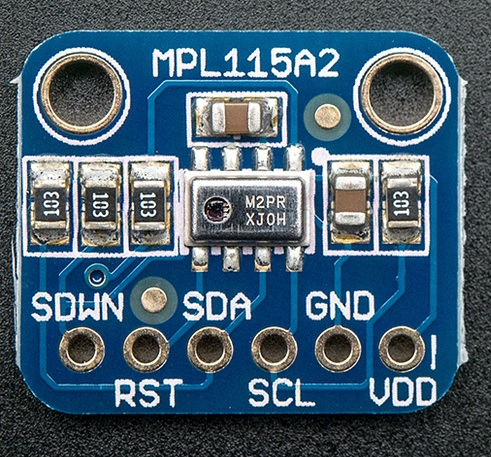float pressure = 0.0;
float temperature = 0.0;
float altitude = 0.0;
float sealevel = 0.0;
sensor.testSensor();
while (!doWork) {
temperature = sensor.getTemperature(true);
pressure = sensor.getPressure(false);
altitude = sensor.getAltitude();
sealevel = sensor.getSealevelPressure();
std::cout << "pressure value = " << pressure << ", altitude value = " << altitude
<< ", sealevel value = " << sealevel << ", temperature = " << temperature
<< std::endl;
upm_delay_us(500000);
}

## Public Member Functions

MPL3115A2 (int bus, int devAddr=MPL3115A2_I2C_ADDRESS, uint8_t mode=6)

int testSensor (void)

int resetSensor (void)

void dumpSensor (void)

int sampleData (void)

int32_t getPressureReg (int reg)

int32_t getTempReg (int reg)

float getPressure (int bSampleData=true)

float getTemperature (int bSampleData=true)

float getSealevelPressure (float altitudeMeters=0.0)

float getAltitude (float sealevelPressure=101325.0)

void setOversampling (uint8_t oversampling)

uint8_t getOversampling (void)

float getTemperatureMax (void)

float getTemperatureMin (void)

float getPressureMax (void)

float getPressureMin (void)

float convertTempCtoF (float fTemp)

float convertPaToinHg (float fPressure)

mraa::Result i2cWriteReg (uint8_t reg, uint8_t value)

uint16_t i2cReadReg_16 (int reg)

uint8_t i2cReadReg_8 (int reg)

## Constructor & Destructor Documentation

 MPL3115A2 ( int bus, int devAddr = `MPL3115A2_I2C_ADDRESS`, uint8_t mode = `6` )

Instantiates an MPL3115A2 object

Parameters
 bus Number of the used bus devAddr Address of the used I2C device mode MPL3115A2 oversampling (6 = 64x)

Here is the call graph for this function: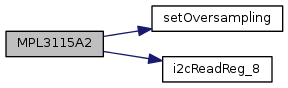## Member Function Documentation

 int testSensor ( void )

MPL3115A2 object destructor; basically, it closes the I2C connection. ~MPL3115A2(); no need for this here, as the I2c connection will be closed when the m_i2ControlCtx variable will go out of scope Tests the sensor and tries to determine if the sensor is operating by looking for small variations in the value

Here is the call graph for this function: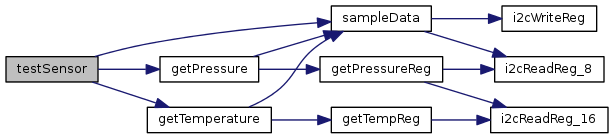int resetSensor ( void )

Performs a soft reset of the MPL3115A2 device to ensure it is in a known state. This function can be used to reset the min/max temperature and pressure values.

Here is the call graph for this function: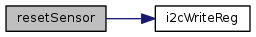void dumpSensor ( void )

Dumps out the I2C register block to stdout

Here is the call graph for this function:int sampleData ( void )

Initiates a temperature/pressure mesasurement and waits for the function to complete. Temperature and pressure registers can be read after this call.

Here is the call graph for this function: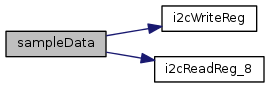Here is the caller graph for this function: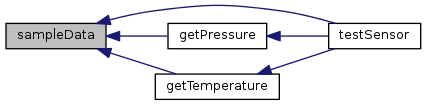int32_t getPressureReg ( int reg )

Reads the pressure value from MPL3115A2 [Pa * 100]

Parameters
 reg Base address of the pressure register

Here is the call graph for this function: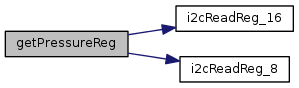Here is the caller graph for this function: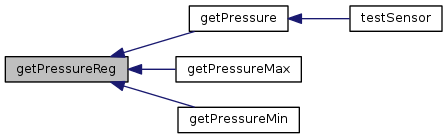int32_t getTempReg ( int reg )

Reads the temperature value from MPL3115A2 [degC * 1000]

Parameters
 reg Base address of the temperature register

Here is the call graph for this function: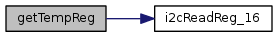Here is the caller graph for this function: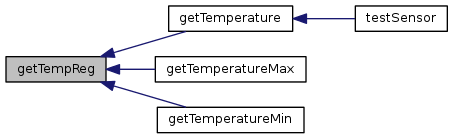float getPressure ( int bSampleData = `true` )

Reads the current pressure value from MPL3115A2 [Pa]

Parameters
 bSampleData Sets non-zero to a sample reading

Here is the call graph for this function: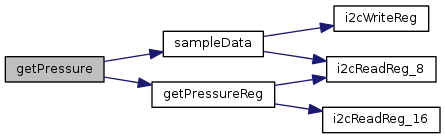Here is the caller graph for this function: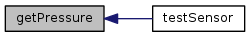float getTemperature ( int bSampleData = `true` )

Reads the current temperature value from MPL3115A2 [degC]

Parameters
 bSampleData Sets non-zero to a sample reading

Here is the call graph for this function: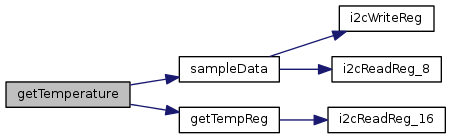Here is the caller graph for this function: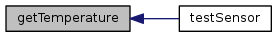float getSealevelPressure ( float altitudeMeters = `0.0` )

Reads the current pressure and, using a known altitude, calculates the sea level pressure value [Pa] This function should be preceded by the sampleData() call

Parameters
 altitudeMeters Altitude in meters
 float getAltitude ( float sealevelPressure = `101325.0` )

Reads the current pressure and, using a known sea level pressure, calculates the altitude value [m] This function should be preceded by the sampleData() call

Parameters
 sealevelPressure Current sea level pressure
 void setOversampling ( uint8_t oversampling )

Defines the oversampling setting (ranges from 0 to 7). The value represents 2^n samples (ranging from 1 to 128). The time to calculate a sample is approximately (2^n * 4 + 2) ms

Parameters
 oversampling New oversampling value

Here is the caller graph for this function: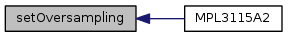uint8_t getOversampling ( void )

Returns the current oversampling value

 float getTemperatureMax ( void )

Reads the maximum measured temperature [degC]

Here is the call graph for this function: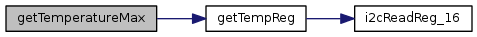float getTemperatureMin ( void )

Reads the minimum measured temperature [degC]

Here is the call graph for this function: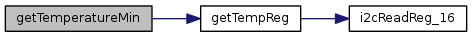float getPressureMax ( void )

Reads the maximum measured pressure [Pa]

Here is the call graph for this function: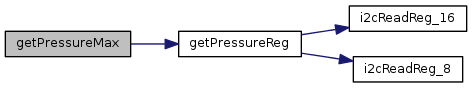float getPressureMin ( void )

Reads the minimum measured pressure [Pa]

Here is the call graph for this function: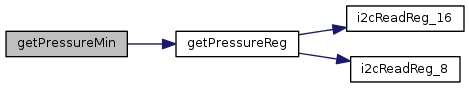float convertTempCtoF ( float fTemp )

Converts temperature from degC*1000 to degF*1000

Parameters
 fTemp Temperature in degC
 float convertPaToinHg ( float fPressure )

Converts pressure from Pa*100 to inHg*10000 This is set for 15degC (Pa = 0.0002961 in Hg) TODO: Change the function to add temperature calibration

Parameters
 fPressure Pressure in Pa
 mraa::Result i2cWriteReg ( uint8_t reg, uint8_t value )

Writes one byte to an I2C register

Parameters
 reg Address of the register value Byte to be written

Here is the caller graph for this function: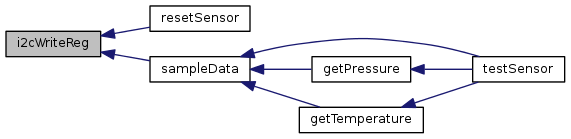uint16_t i2cReadReg_16 ( int reg )

Reads two bytes from an I2C register

Parameters
 reg Address of the register

Here is the caller graph for this function: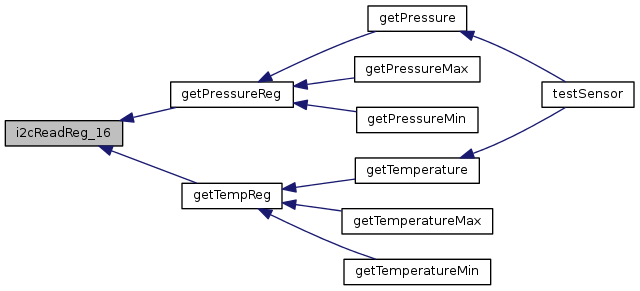uint8_t i2cReadReg_8 ( int reg )

Reads a one-byte register

Parameters
 reg Address of the register

Here is the caller graph for this function: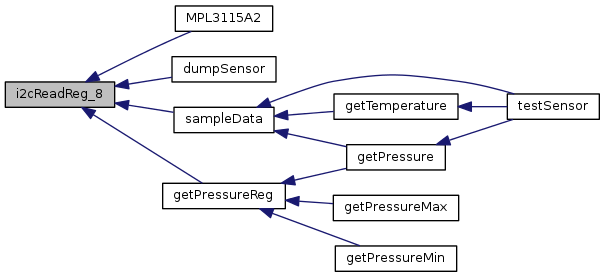The documentation for this class was generated from the following files: